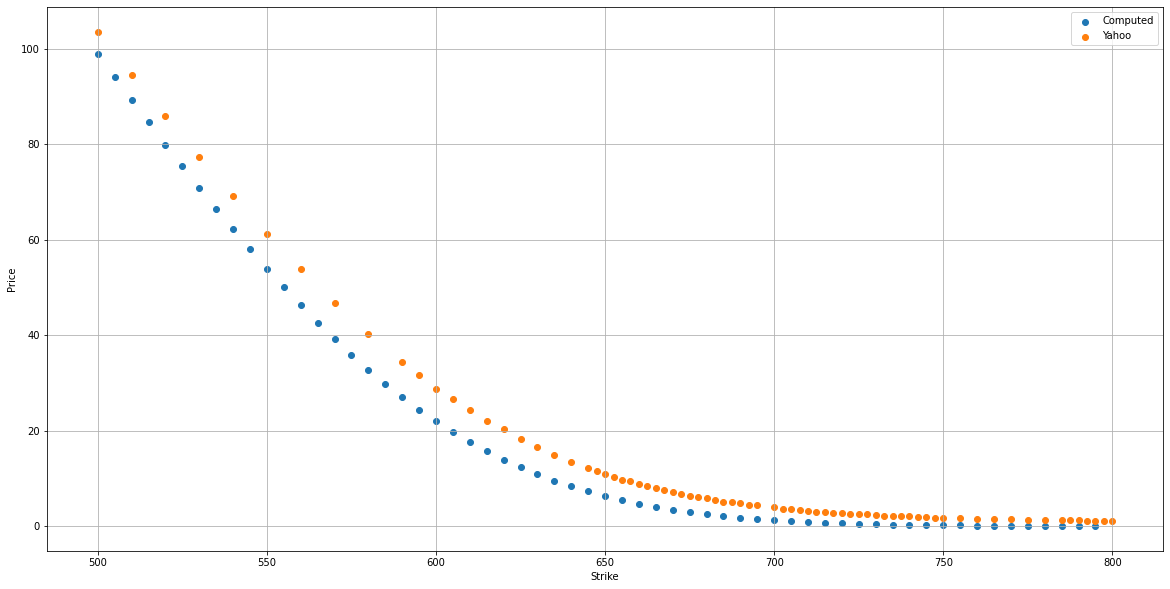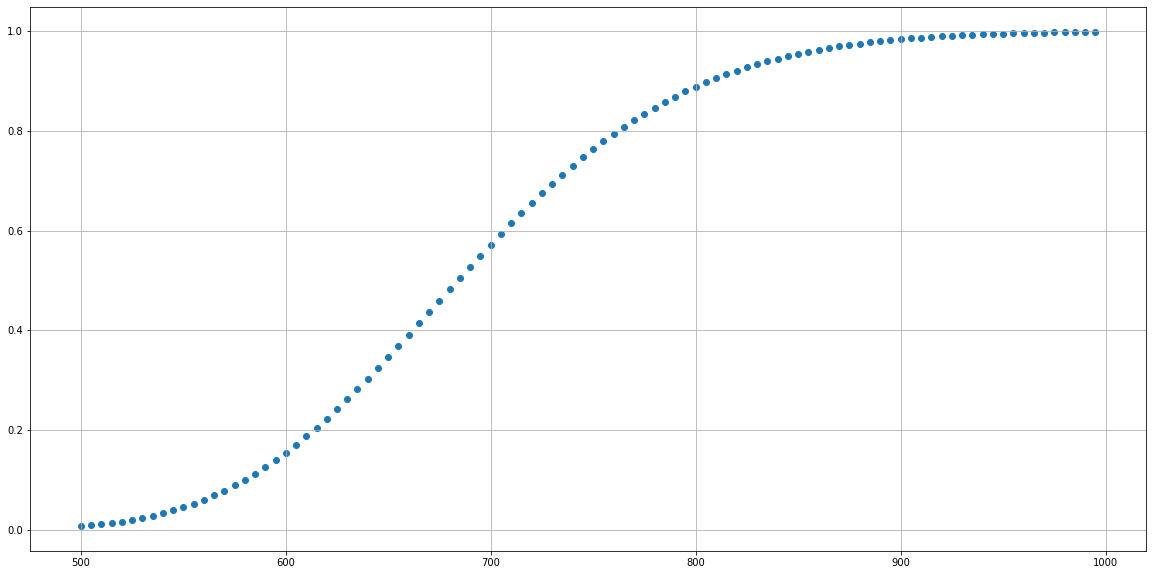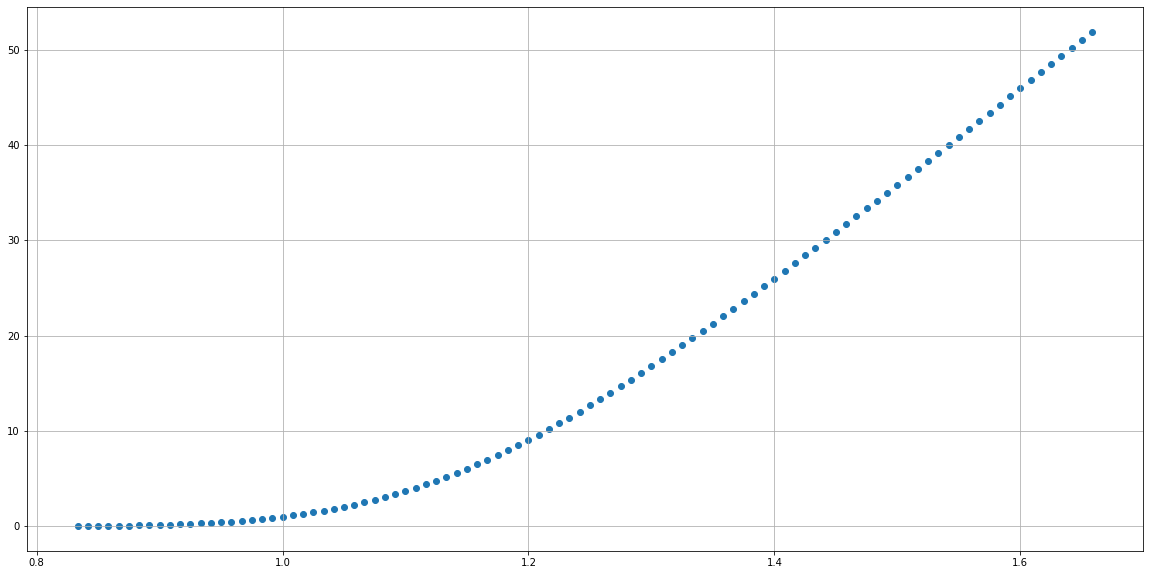# Option Pricing Model

The price of option depends on many factors, current stock price, strike price, implied volatility and expiration date. According to Investopedia, the most common pricing model is Black Scholes Model. Howerver, it does not take into account the execution of American Style options, which can be exercised at any time before, and including the day of, expiration, for which Binomial Option Pricing Model should be used.

# Black Scholes Model

The model assumes the price of heavily traded assets follows a geometric Brownian motion with constant drift and volatility. When applied to a stock option, the model incorporates the constant price variation of the stock, the time value of money, the option’s strike price, and the time to the option’s expiry.

The Black-Scholes model makes certain assumptions:

• The option is European and can only be exercised at expiration.
• No dividends are paid out during the life of the option.
• Markets are efficient (i.e., market movements cannot be predicted).
• There are no transaction costs in buying the option.
• The risk-free rate and volatility of the underlying are known and constant.
• The returns on the underlying asset are normally distributed.

The Black Scholes call option formula is calculated by multiplying the stock price by the cumulative standard normal probability distribution function.

\begin{align*} C&=S_t \mathcal{N}(d_1)-Ke^{-rt}\mathcal{N}(d_2) \\ d_1&=\frac{\ln{\frac{S_t}{K}}+(r+\frac{\sigma^2}{2})t}{\sigma\sqrt{t}} \\ d_2&=d_1-\sigma\sqrt{t} \end{align*}

where

• C=Call option price
• S=Current stock (or other underlying) price
• K=Strike price
• r=Risk-free anual interest rate
• t=Years to maturity
• $$\mathcal{N}$$ =cumulative density function of normal distribution
• $$\sigma$$ =annaul volalicity of the stock which is relative change of price within one year

the Black Scholes model is only used to price European options and does not take into account that U.S. options could be exercised before the expiration date

## Implementations

I have implemented this model in colab:

import sympy
from sympy import ln, exp, symbols, sqrt, pi
from sympy.stats import Normal, cdf

sympy.init_printing()

def normal(x):
return exp(- (x ** 2) / 2) / sqrt(2 * pi)

def normal_cdf(x):
return sympy.simplify(cdf(Normal("xx", 0, 1)))(x)

def option_price(
years_to_expire: float,
current_price: float,
strike_price: float,
volatility: float,
anual_interest_rate: float=0.04):
d1 = (ln(current_price / strike_price) + (anual_interest_rate + volatility ** 2 / 2) * years_to_expire) / (volatility * sqrt(years_to_expire))
# print(sympy.latex(d1))
d2 = d1 - volatility * sqrt(years_to_expire)
# print(sympy.latex(d2))
price = current_price * normal_cdf(d1) - strike_price * exp(- anual_interest_rate * years_to_expire) * normal_cdf(d2)
# print(sympy.latex(price))
return price

t, s, k, sigma, r = symbols("t, s, k, sigma, r")
price = option_price(t, s, k, sigma, r)


## Option Price vs Strike Price

Let us validate it for Tesla call option expires on 3/12/2021 and this computation is done at 3/7/2021, thus we have s=598, t=5.0/365, r=0.04 and sigma=0.816 (using data here):

$- 0.5 k \left(\operatorname{erf}{\left(\sqrt{2} \left(5.235 \log{\left(\frac{598}{k} \right)} - 0.021\right) \right)} + 1\right) + 299 \operatorname{erf}{\left(\sqrt{2} \left(5.235 \log{\left(\frac{598}{k} \right)} + 0.0267\right) \right)} + 299$

This plot shows a comparison of prices computed with this code vs Yahoo’s data from strike price from 500 to 800, which shows a decent match:If we change the sigma from 0.816 to 1.1, we could get a much better alignment. That means option pricing expect a very high volatility for Tesla in near future.Here is the code

current_price = 598
years_to_expire = 5.0 / 365
annual_interest_rate = 0.04
volatility = 1.1
price_to_strike = price.subs({s: current_price, t: years_to_expire, r: annual_interest_rate, sigma: volatility})
price_to_strike = sympy.simplify(price_to_strike)

strike_price = numpy.arange(500, 800, 5)
evaluated_price = [price_to_strike.subs({k: sp}) for sp in strike_price]
pyplot.scatter(strike_price, evaluated_price)


## Option Price vs Stock Price

Now let us look at how the option price changes with the stock price as of now. Let us still consider Tesla call option on 3/12/2021, use k=690, t=5.0/365, r=0.04 and sigma=0.816. Here is a plot for option price vs stock price.Here is the code:

strike_price = 690
price_to_current = price.subs({k: strike_price, t: years_to_expire, r: annual_interest_rate, sigma: volatility})
price_to_current = sympy.simplify(price_to_current)
print(sympy.latex(price_to_current))

current_price = numpy.arange(500, 800, 5)
evaluated_price = [price_3_12.subs({s: cp}) for cp in current_price]
pyplot.scatter(current_price, evaluated_price)
pyplot.ylabel("Current Price")
pyplot.xlabel("Strike")
pyplot.grid(True)


Or look at gradient we have this plot, which indicates that as the current stock price increases, the corresponding option price will also increase but much slower first and then converges to the same change as stock price.Here is the code

gradient_price = sympy.diff(price, s)
strike_price = 690
current_price = numpy.arange(500, 1000, 5)
evaluated_price = [gradient_price.subs({s: cp}) for cp in current_price]
pyplot.scatter(future_price, evaluated_price)


However, if we look at relative change on stock price vs relative change on option price, we have the following plot. This could explain why people want to look into option for higher return rate, e.g., when stock price increases 20% from 600 to 720, the option price increases by almost 20 times.Here is the code:

# consider relative change between stock price and option price
base_stock_price = 600
base_option_price = price_to_current.evalf(subs={s: base_stock_price})

future_price = numpy.arange(500, 1000, 5)
evaluated_price = [price_to_current.evalf(subs={s: ss}) / base_option_price for ss in future_price]
fig = pyplot.scatter(future_price / base_stock_price, evaluated_price)
fig.axes.grid(True, which="both")


# Binomial Option Pricing Model

The binomial option pricing model uses an iterative procedure, allowing for the specification of nodes, or points in time, during the time span between the valuation date and the option’s expiration date. It could handle America option, where you could excersize your option before expiration.

With binomial option price models, the assumptions are that there are two possible outcomes: a move up, or a move down. The portfolio value remains the same regardless of which way the underlying price goes, the probability of an up move or down move does not play any role. The portfolio remains risk-free regardless of the underlying price moves, otherwise the buyers and sellers will not balance.

For example, current stock price is 100. There is 50/50 chance the stock price will increase or decrease by 10 next period. Assume an investor buy $$d$$ share of stock and write/sell on call option with strike price of 100, then:

• cost today 100d - option price
• portfolio value in next period:
• go up: $$110d - \max{(110-100,0)}$$
• go down: $$90d - \max{(90-100,0)}$$
• to have two portfolio value be equal, we have $$110d-10=90d=45$$ where d = 0.5

Then the option price is computed as:

$option\ price=50-45\times e^{-rT}$

Assuming the risk-free rate is 3% per year, and T equals 0.0833 (one divided by 12), then the price of the call option today is \$5.11.

Please check Understanding the Binomial Option Pricing Model for more details.

Written on February 7, 2021